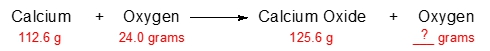## General Chemistry

Learn the toughest concepts covered in Chemistry with step-by-step video tutorials and practice problems by world-class tutors

2. Atoms & Elements

# Law of Conservation of Mass

"In a chemical reaction, no matter is created or destroyed, but instead changes form."

Lavoisier's Conservation of Mass
1
concept

## Law of Conservation of Mass1m
Play a video:
In 17 89 the French chemist Antoine of a sore, who's credited as being another father of chemistry, originated the law of Conservation of Mass. Now the law states that in a chemical reaction, no matter is created or destroyed, but instead changes form in a chemical reaction. We're going to say that the compounds before the arrow so in this case H 202 would be are reacting. It's and those after the arrow. In this case, h 20 would be our products, according to Labus, or all of the reactant seats are converted to product with nothing lost. So to think about it, we could say that originally at the beginning of the reaction, we have H two and 02 and mixing them together their combined a map of both of them together. Let's say it's 100 g, while according to the law of conservation of Mass, nothing is truly lost is just converted from one form to another, so all 100 g of my reactions should be converted entirely into product. So at the end I have exactly 100 g of product. All of this would be gone. All of this has been transformed into hte So what this tells me is that if you know the total massive air reactions in the beginning, then they should equal the total mass of products that you create at the end. So this is an incredibly important idea when it comes to the law of conservation of Mass that will connect to other ideas later on, such as soy geometry and solution chemistry. So just remember, when it comes to law of conservation of mass, it helps us to determine eventually the amount of product we could potentially form.

According to Lavoisier, all reactants are completely converted into products.

2
example

## Law of Conservation of Mass Example 11m
Play a video:
in this example question. It says how many grams of water vapor will form if 25 brands of hydrogen gas mixes with 12 g of oxygen gas. Now, applying what we just learned about the law of conservation of Mass. This is gonna be too bad, because remember, it says that the mass, the total mass of reactant, will equal the total mass of products. Here we have the masses of the reactions, which are 25 12. So we add them together. That will give us the total mass of product that could potentially form. So this answer here would be 37 g of products. Now, if we want to follow the law on the rules for significant figures, Technically, this has three sig figs, and this has three sig figs. So the better answer would be 37.0 g of water vapor, which is just water as a gas. So just remember, if we're just simply applying the law of conservation of Mass, just remember the amount of reacting Sequels of my amount of products. Now, later on, when we go into more advanced forms of calculations with chemical reactions, we're going to see, that's not always true. In those cases, we learn new mechanics and new approaches to enter those particular type of questions.
3
Problem

Following the Law of Conservation of Mass, predict the minimum amount of nitrogen that will react with 50.0 grams of hydrogen to produce 92.5 grams of ammonia.

Nitrogen + Hydrogen → Ammonia

4
Problem

Predict the amount of oxygen gas that will remain after the reaction of 112.6 grams of calcium with 24.0 grams of oxygen.